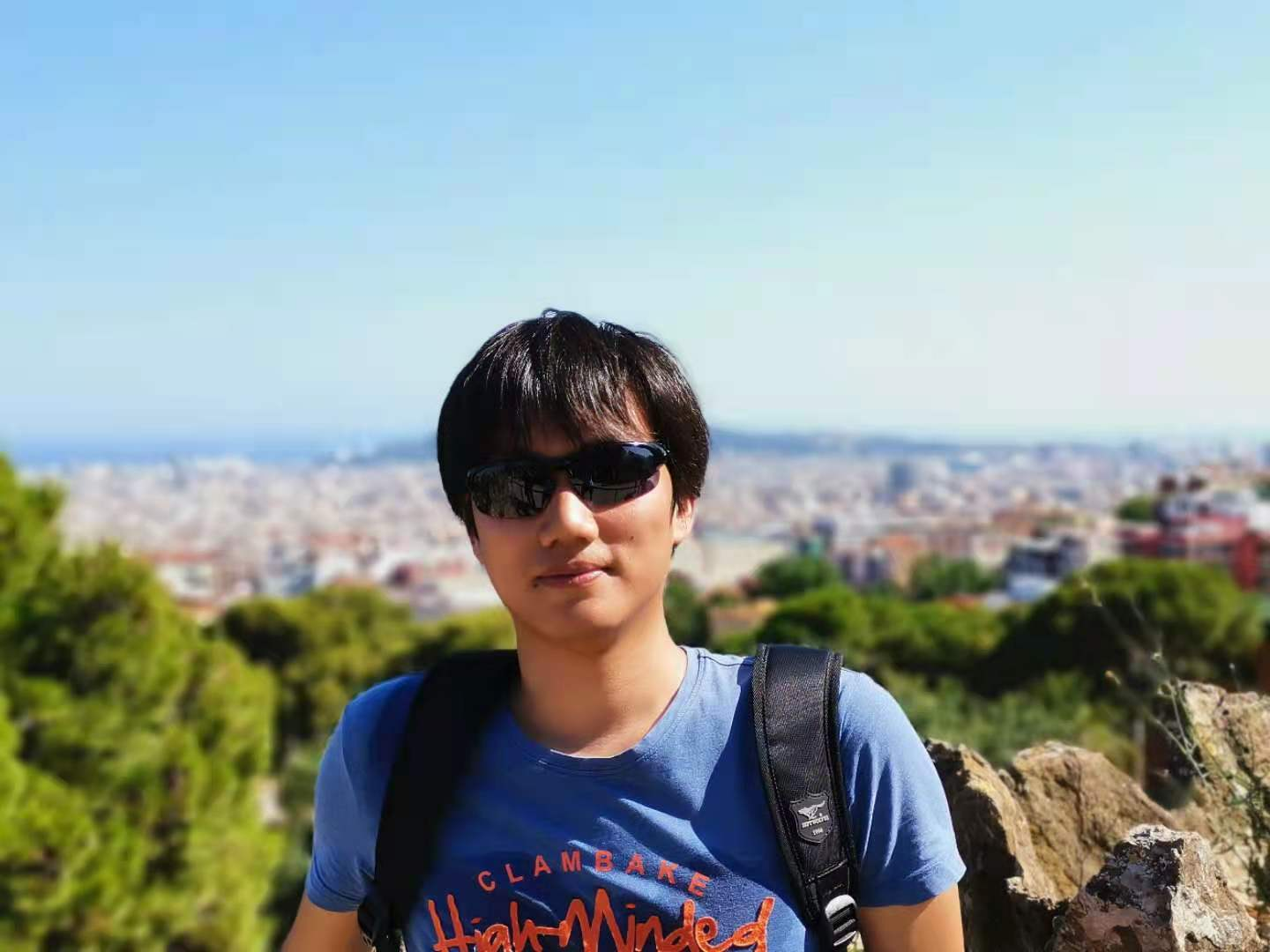# For: Local and Central Differential Privacy for Robustness and Privacy in Federated Learning

10206

## 背景及简介

• **污染攻击 (Poisoning Attacks)**：泛指用于降低模型预测的准确率或者让模型在某些特定的输入上给出错误的预测的攻击类型
• 后门攻击：恶意用户将后门注入到训练的模型中，让模型在某些情况下给出攻击者想要的预测结果
• 成员推断攻击：用于预测某个样本是否在训练集中
• 属性推断攻击：用于预测样本的某个与训练任务无关的属性（比如在某个图片性别分类器的训练过程中，判断某个人是否长了胡子）

• 本地DP（Local Differential Privacy, LDP）：FL参与者将模型更新发送给服务器之前添加噪声
• 中心DP（Central Differential Privacy, CDP）：服务器使用DP保护的聚合算法

## FL的差分隐私保护方案

### 本地DP

LDP 即FL的非中心化节点对本地的梯度更新加入噪声，而后才将地图更新发送给中心化服务器进行聚合。文中提到的LDP主要有两个手段：

1. 使用二范数对梯度进行截断，即$g = g \cdot \min(1, \cfrac{S}{\Vert g\Vert_2})$，其中$g$为原本的梯度值，$S$为预设定阈值
2. 加入高斯噪声，即$g = g + N(0, \sigma^2I)$，其中$N$为高斯分布

### 中心DP

CDP即聚合服务器在模型/梯度聚合的过程中引入噪声，旨在保护参与者的隐私（让攻击者无法判断某个参与者是否参与了训练过程）。CDP方案也使用了二范数对参与者的梯度进行截断，并且对于高斯噪声方差的选择使用了论文中的计算方法，感兴趣可查看原论文：$\sigma = \cfrac{z\cdot S}{q}$，其中$S$为阈值，$q$为希望引入的噪声比例，$q$为参与训练人员的采样概率。

## 后门攻击测量分析

$$\Delta\theta_\mathcal{A} = \cfrac{\sum_{i=1}^{N}n_i}{n_\mathcal{A}} \cdot(\theta_\mathcal{A} - \theta_r)$$

\begin{align} \Delta\theta_{r+1} &= \cfrac{\sum_{i=1}^{N} n_i \cdot \Delta{\theta_{i}}}{\sum_{i=1}^{N} n_i }\\ & = \cfrac{n_\mathcal{A} \cdot \Delta \theta_\mathcal{A} + \sum_{i\ne\mathcal{A}}n_i \cdot \Delta\theta_i}{\sum_{i=1}^{N} n_i} \\ & \approx \cfrac{n_\mathcal{A} \cdot \Delta \theta_\mathcal{A}}{\sum_{i=1}^{N} n_i} & ; 假设在训练末期，正常梯度都近似为0\\ & = \theta_\mathcal{A} - \theta_{r} \end{align}

### 实验测量

1. EMNIST
2. CIFAR10
4. Sentiment140

1. 单像素后门攻击（EMNIST数据集）：将手写数字图片的右下角的一个像素点改为0值，让模型预测此图片为手写$0$
2. 语义后门攻击（CIFAR10）：让特定模式的图片被预测为某个分类，如：让红色的图片被预测为猫
4. 语义后门攻击（Sentiment140）：让情感分析的模型将I feel great分类为消极情绪

## 推断攻击测量分析

### 成员推断攻击

FL场景下的攻击稍微复杂一点，攻击者不但能做成员推断，而且可以推断某个样本是否来自某个客户端。此外，攻击者可以为中心服务器，也可以是单个训练的客户端。中心化服务器的攻击方式更为多样，比如它可以将单个用户隔离开来（使用单独的一个模型），这样模型中就可以存储更多的用户数据信息。

#### 实验测量及结果

1. CIFAR100
2. Purchase100：消费者在线购买记录
3. Texas100：医疗记录

## 主要结论

1. LDP 和 CDP 在牺牲性能的前提下都可以在某种程度上防御后门攻击
2. 如果仅对非攻击者应用LDP，那么后门攻击的准确率会提升
3. LDP和CDP都可在不引入太大性能损耗的前提下防御成员推断攻击
4. LDP对属性推断攻击无能为力；虽然理论上CDP可以防御属性推断攻击，但是性能损失的代价非常大

## 引用

 Naseri, Mohammad, Jamie Hayes, and Emiliano De Cristofaro. "Local and central differential privacy for robustness and privacy in federated learning."arXiv preprint arXiv:2009.03561(2020).

 Abadi, Martin, et al. "Deep learning with differential privacy."Proceedings of the 2016 ACM SIGSAC conference on computer and communications security. 2016.

Article Tags
[本]通信工程@河海大学 & [硕]CS@清华大学

0
10206
0

More RecommendationsJuly 18, 2022June 15, 2022June 8, 2022June 1, 2022